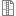# Visualizing Wrong Vertices of the Selected Surface

Hello OCCT Community,

After reading the STEP file, I made an implementation to show each corner and center point when I click on any face on the resulting shapes. In some STEP files this works, in others it draws the vertex out of the shape. This problem can be seen in the images that I have attached. And, I'm sharing my code-snippets:

When mouse pressed:

``````        if(!ais_interactive_context_->DetectedOwner().IsNull())
{
std::cout<<"Detected Owner of AIS Interactive Context is not NULL!"<<std::endl;
Handle(AIS_InteractiveObject) picked = ais_interactive_context_->DetectedInteractive();
ais_interactive_context_->InitSelected();
Handle(SelectMgr_EntityOwner) aSelOwner  = ais_interactive_context_->SelectedOwner();
Handle(SelectMgr_EntityOwner) detectedOwner = ais_interactive_context_->DetectedOwner();
Handle(StdSelect_BRepOwner)   aBRepOwner = Handle(StdSelect_BRepOwner)::DownCast (detectedOwner);
if (!aBRepOwner.IsNull() && aBRepOwner->Shape().ShapeType() == TopAbs_FACE){
face_sub_shape_ =  TopoDS::Face(aBRepOwner->Shape());
std::cout<<"Selected BRepOwner is not NULL & Shape Type is FACE!"<<std::endl;
}else if(!aBRepOwner.IsNull() && aBRepOwner->Shape().ShapeType() == TopAbs_VERTEX){
vertex_sub_shape_ =  TopoDS::Vertex(aBRepOwner->Shape());
std::cout<<"Selected BRepOwner is not NULL & Shape Type is VERTEX!"<<std::endl;
std::cout<<"Selected Shape type is VERTEX! "<<std::endl;
for(TopExp_Explorer vertEx(vertex_sub_shape_, TopAbs_VERTEX); vertEx.More(); vertEx.Next()) {
TopoDS_Vertex aVertex = TopoDS::Vertex(vertEx.Current());
gp_Pnt aPnt = BRep_Tool::Pnt(aVertex);
std::cout << "Selected VERTEX of the Selected FACE: " << aPnt.X() << " " << aPnt.Y() << " " << aPnt.Z()<<std::endl;
this->createSphere(aPnt, 5.0, Quantity_NOC_RED);
}
}else {
std::cout<<"Selected BRepOwner is NULL!"<<std::endl;
return;
}

if ( face_sub_shape_.ShapeType() == TopAbs_FACE )
{
const TopoDS_Face& face = TopoDS::Face(face_sub_shape_);
GProp_GProps surfaceProperties;
BRepGProp::SurfaceProperties(face_sub_shape_,surfaceProperties, false, false);
std::cout << "Surface's Center of Mass: " << surfaceProperties.CentreOfMass().X() << " " << surfaceProperties.CentreOfMass().Y() << " " << surfaceProperties.CentreOfMass().Z() << std::endl;
gp_Pnt center_point_of_surface (surfaceProperties.CentreOfMass().X(), surfaceProperties.CentreOfMass().Y(), surfaceProperties.CentreOfMass().Z());
this->createVertex(center_point_of_surface, Quantity_NameOfColor::Quantity_NOC_BLUE);

// Show all Vertices of the Selected Surface
for(TopExp_Explorer vertEx(face_sub_shape_, TopAbs_VERTEX); vertEx.More(); vertEx.Next()) {
TopoDS_Vertex aVertex = TopoDS::Vertex(vertEx.Current());
gp_Pnt aPnt = BRep_Tool::Pnt(aVertex);
this->createVertex(aPnt, Quantity_NameOfColor::Quantity_NOC_BLUE);
}
}
}
updateView();
}
``````

Do you have any suggestion about this issue? Could you clearly understand where I made a mistake when you look at the code? If not, I can add more code snippets.

Attachments:Vertices on the shape.pngVertices outside the shape.pngVerticesOutsideTheShape-SampleStepFile.7zVerticesOnTheShape-SampleStepFiles.7zHow shape is displayed in the viewer? Maybe your AIS_Shape has non-identity location?``````...
Handle(AIS_Shape) entire_ais_shape_;
...
``````
``````void MyVisualizerWidget::setInteractiveContext(MyMeshModel named_solid)
{

entire_ais_shape_ = named_solid.getMeshObject();
shape_map_.emplace(named_solid.getMeshModelId(), entire_ais_shape_);
entire_ais_shape_->SetLocalTransformation(named_solid.getMeshLocation());

if (named_solid.getMeshObject())
{
ais_interactive_context_->Activate(entire_ais_shape_, entire_ais_shape_->SelectionMode( TopAbs_FACE));
ais_interactive_context_->Activate(entire_ais_shape_, entire_ais_shape_->SelectionMode( TopAbs_VERTEX));
}
}
````````````void MyVisualizerWidget::createVertex(gp_Pnt vertex_coordinate, Quantity_NameOfColor vertex_color)
{
TopoDS_Vertex topods_vertex = BRepBuilderAPI_MakeVertex(gp_Pnt(vertex_coordinate.X(), vertex_coordinate.Y(), vertex_coordinate.Z())).Vertex();
Handle_AIS_Shape ais_vertex_shape = new AIS_Shape(topods_vertex);
ais_vertex_shapes_.push_back(ais_vertex_shape);
ais_vertex_shape->SetColor(vertex_color);
ais_interactive_context_->Display(ais_vertex_shape, true);
}

void MyVisualizerWidget::showSelectedVertices()
{
for(auto v: vertices_of_selected_face_){
TopoDS_Vertex topods_vertex = BRepBuilderAPI_MakeVertex(gp_Pnt(v.X(), v.Y(), v.Z())).Vertex();
Handle_AIS_Shape ais_vertex_shape = new AIS_Shape(topods_vertex);
ais_vertex_shape->SetColor(Quantity_NOC_ALICEBLUE);
ais_vertex_shapes_.push_back(ais_vertex_shape);
ais_interactive_context_->Display(ais_vertex_shape, true);
}
}
``````entire_ais_shape_->SetLocalTransformation(named_solid.getMeshLocation());

So indeed, you assign transformation to AIS_Shape presentation. TopoDS_Shape returned by StdSelect_BRepOwner::Shape() usually doesn't include this transformation - so that you may try to apply this location (your STEP file does define some transformations to shapes).Hello Kirill,

I've been investigating and trying on official OCCT samples with some modifications for a while. For example, I've used the "STEP import methods from OCCT/samples/ios/UIKitSample/UIKitSample" for getting individual labels in STEP topology and list them in a tree on UI. This application is attached as sample_1.zip. The main problem here is that when I select a face, it places the vertices in an area outside the object (Just like the problem I mentioned before, but this time I'm adding the whole sample program here for a clearer understanding).

As another example I've used the "OCCT/samples/qt/OCCTOverview --> Data Exchange Category & Import STEP Sample" and in this example, whichever face I clicked, the vertices on it visualizes and this was exactly the result I would like to achieve. What I require to add here is to take each label in the step topology separately, as I mentioned in the first example, and list them in a tree. So if I visualize the assembly as a whole and select it with the mouse, it correctly positions the vertexes. This application also is attached as sample_2.zip.

I apologize for could not understanding your solution. However, do I need to go to the solution without using the SetLocalTransformation method here? If so, how can I properly visualize the location information I get while reading the STEP file?sample_1.zipsample_2.zipOne object- True location of vertices.pngwrong vertex locations of selected face.PNG